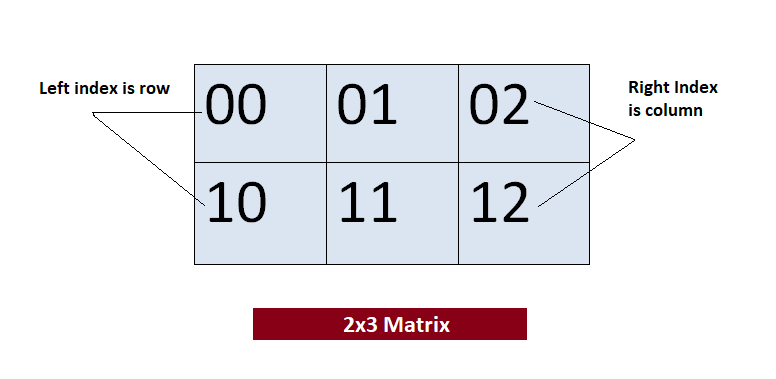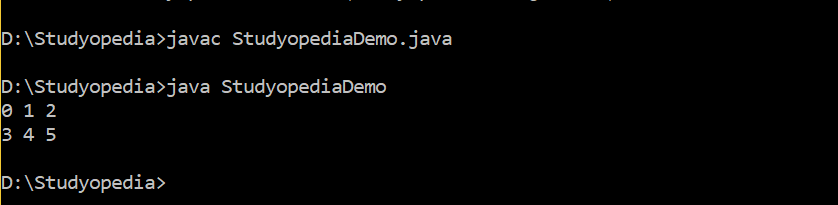## 09 May Multidimensional Arrays in Java

In the last lesson, we learned about One-Dimensional Arrays in Java and how to use them. Now, we will see about a concept of arrays in Java i.e. Multidimensional Arrays. Multi-dimensional arrays as the name suggests are arrays of arrays. You need to use two square brackets to declare a multidimensional array, unlike one-dimensional array.

Let us see an example to declare a 2 by 3 multidimensional array,

The following figure displays how a multidimensional array forms a 2×3 matrix,Above, the left index determines row and the right index determines column.

We will now see how to display each element from our 2×3 matrix,

The following is the output displaying each element,In this lesson, we saw how to work with Multidimensional arrays in Java.

##### Tags:[email protected]

We work to create programming tutorials for all.